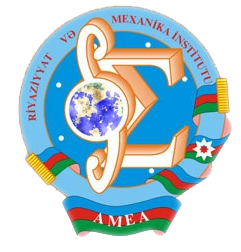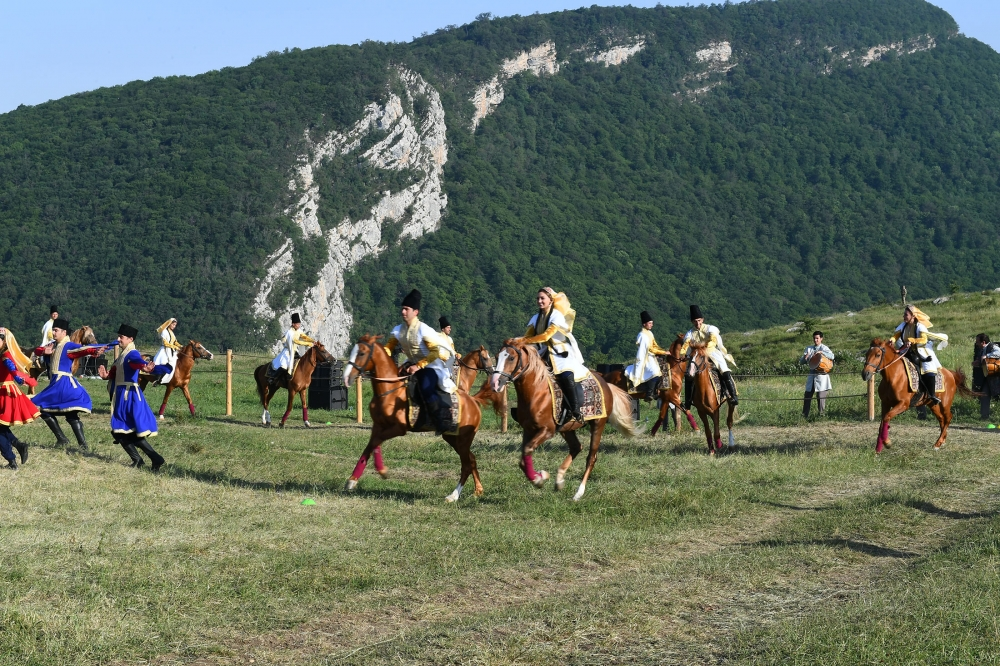Riyaziyyat və Mexanika İnstitutu

## Qarabağ xəbərləri### Şuşada Heydər Əliyev Fondunun təşkilatçılığı ilə “Musiqi irsi və Qarabağ atları Cıdır düzündə” adlı kompozisiya təqdim olunub## Proceedings 2012/36/XLIV

 Year: 2012 Volume: XXXVI Number: XLIV MATHEMATICS Abdullayev F.A., Gasymova N.F. Abstract PDF On solution of a class of nonlinear singular integral equations with cauchy kernel [PDF] 3 Azizov F.J. Abstract [PDF] On asymptotic behavior of conditional probability of crossing the nonlinear boundary by Markov chain trajectory [PDF] 9 Badalova K.G., Aslanov G.I. Abstract [PDF] Weighted trace of higher order operator-differential equations on the semi-axis [PDF] 17 Dostuyev F.Z. Abstract [PDF] Properties of eigen values of sturm-liouville periodic and antiperiodic operators with discontinuity conditions [PDF] 25 Garayeva A.T. Abstract [PDF] On absolute convergence of expansions in eigen vector-functions of sturm-liouville operator with matix coeffcient [PDF] 31 Gonul N., Coshkun E. Abstract [PDF] Weighted approximation by positive linear operators [PDF] 41 Imanova A.B. Abstract [PDF] Angular boundary values of poisson integral in terms of mean oscilation [PDF] 51 Isayev F.A., Safarov Z.V. Abstract [PDF] Weighted inequality for singular integrals in Lebesgue spaces, associated with the Laplace-Bessel differential operators [PDF] 61 Mamedova K.N. Abstract [PDF] On behavior of solutions of higer order degeenrate parabolic equations in nonsmooth bounded domains [PDF] 69 Mehraliyev Y.T. Abstract [PDF] An inverse boundary value problem for a fourth order elliptic equation [PDF] 75 Osmanly J.A. Abstract [PDF] On the properties of passage coe±cient of schrodinger one-dimensional equation with discontinuity conditions [PDF] 91 MECHANICS Mikailova N.M. Abstract [PDF] Vibrations of viscoelastic membrane [PDF] 97 Nagiyeva N.M. Abstract [PDF] On a characteristic function of fatigue failure under asymmetric loading cycles [PDF] 105 APPLIED PROBLEMS OF MATHEMATICS AND MECHANICS Guliyev H.F., Zeynalli S.M. Abstract [PDF] On application of optimal control methods to the solution of the cauchy problem for a second order elliptic equation [PDF] 109 Mustafayeva T.M. Abstract [PDF] An optimal control problem for a hyperbolic equation with phase restriction [PDF] 117 Sharifov Y.A. Abstract [PDF] Existence and uniqueness of solutions for nonlinear fractional differential equations with nonlocal boundary conditions [PDF] 125 Tagiyev H.T. Abstract [PDF] On existence of solution on nonlocal condition optimal control problem for wave equation [PDF] 135
Azərbaycanda COVID-19 ilə bağlı statistika
• Virusa yoluxan

596973

• Sağalan

565521

• Yeni yoluxan

0

• Aktiv xəstə

23469

• Ölüm halı

7983

• Test edilib

5,608,158